Main Content

# HDL Implementation of a Digital Down-Converter for LTE

This example shows how to design a digital down-converter (DDC) for radio communication applications such as LTE, and generate HDL code with HDL Coder™.

### Introduction

DDCs are widely used in digital communication receivers to convert Radio Frequency (RF) or Intermediate Frequency (IF) signals to baseband. The DDC operation shifts the signal to a lower frequency and reduces its sampling rate to facilitate subsequent processing stages. The DDC presented here performs complex frequency translation followed by sample rate conversion using a 4-stage filter chain. The example starts by designing the DDC with DSP System Toolbox™ functions in floating point. Each stage is then converted to fixed-point, and then used in a Simulink® model which generates synthesizable HDL code. Two test signals are used to demonstrate and verify the DDC operation:

1. A sinusoid modulated onto a 32 MHz IF carrier.

2. An LTE downlink signal with a bandwidth of 1.4 MHz, modulated onto a 32 MHz IF carrier.

The example measures signal quality at the output of the floating-point and fixed-point DDCs, and compares the two. Finally, FPGA implementation results are presented.

Note: This example uses `DDCTestUtils`, a helper class containing functions for generating stimulus and analyzing the DDC output. See the `DDCTestUtils.m` file for more info.

### DDC Structure

The DDC consists of a Numerically Controlled Oscillator (NCO), a mixer, and a decimating filter chain. The filter chain consists of a CIC decimator, CIC gain correction, a CIC compensation decimator (FIR), a halfband FIR decimator, and a final FIR decimator. The overall response of the filter chain is equivalent to that of a single decimation filter with the same specification, however, splitting the filter into multiple decimation stages results in a more efficient design which uses fewer hardware resources. The CIC decimator provides a large initial decimation factor, which enables subsequent filters to work at lower rates. The CIC compensation decimator improves the spectral response by compensating for the CIC droop while decimating by two. The halfband is an intermediate decimator while the final decimator implements the precise `Fpass` and `Fstop` characteristics of the DDC. Due to the lower sampling rates, the filters nearer the end of the chain can optimize resource use by sharing multipliers. A block diagram of the DDC is shown below.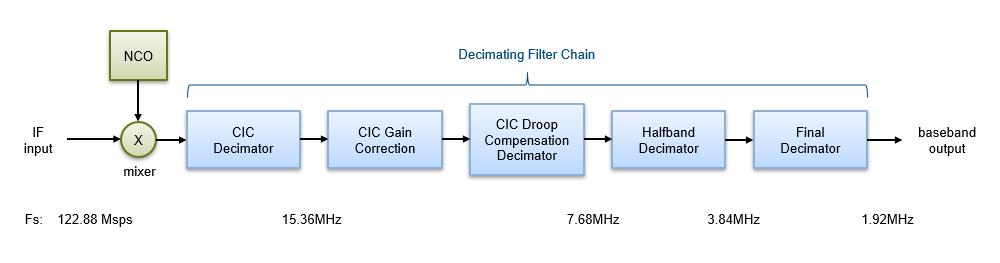The input to the DDC is sampled at 122.88 Msps while the output sample rate is 1.92 Msps. Therefore the overall decimation factor is 64. 1.92 Msps is the typical sampling rate used by LTE receivers to perform cell search and MIB (Master Information Block) recovery. The DDC filters have therefore been designed to suit this application. The DDC is optimized to run at a clock rate of 122.88 MHz.

### DDC Design

This section explains how to design the DDC using floating-point operations and filter-design functions in MATLAB®.

DDC Parameters

The desired DDC response is defined by the input sampling rate, carrier frequency, and filter characteristics. Modifying this desired filter response may require changes to the HDL Block Properties of the filter blocks in the Simulink model. HDL Block Properties are discussed later in the example.

```FsIn = 122.88e6; % Sampling rate at input to DDC Fc = 32e6; % Carrier frequency Fpass = 540e3; % Passband frequency, equivalent to 36x15kHz LTE subcarriers Fstop = 700e3; % Stopband frequency Ap = 0.1; % Passband ripple Ast = 60; % Stopband attenuation ```

The rest of this section shows how to design each filter in turn.

Cascade Integrator-Comb (CIC) Decimator

The first filter stage is implemented as a CIC decimator because of its ability to implement a large decimation factor efficiently. The response of a CIC filter is similar to a cascade of moving average filters, however no multiplies or divides are used. As a result, the CIC filter has a large DC gain.

```cicParams.DecimationFactor = 8; cicParams.DifferentialDelay = 1; cicParams.NumSections = 3; cicParams.FsOut = FsIn/cicParams.DecimationFactor; cicFilt = dsp.CICDecimator(cicParams.DecimationFactor,... cicParams.DifferentialDelay,cicParams.NumSections) %#ok<*NOPTS> cicGain = gain(cicFilt) ```
```cicFilt = dsp.CICDecimator with properties: DecimationFactor: 8 DifferentialDelay: 1 NumSections: 3 FixedPointDataType: 'Full precision' cicGain = 512 ```

The CIC gain is a power of two, therefore it can be easily corrected for in hardware with a shift operation. For analysis purposes, the gain correction is represented in MATLAB by a one-tap `dsp.FIRFilter` System object.

```cicGainCorr = dsp.FIRFilter('Numerator',1/cicGain) ```
```cicGainCorr = dsp.FIRFilter with properties: Structure: 'Direct form' NumeratorSource: 'Property' Numerator: 0.0020 InitialConditions: 0 Use get to show all properties ```

Use `fvtool` to display the magnitude response of the CIC filter with and without gain correction. For analysis, combine the CIC filter and the gain correction filter into a `dsp.FilterCascade` System object. CIC filters always use fixed-point arithmetic internally, so `fvtool` plots both the quantized and unquantized responses.

```ddcPlots.cicDecim = fvtool(... cicFilt,... dsp.FilterCascade(cicFilt,cicGainCorr), ... 'Fs',[FsIn,FsIn]); DDCTestUtils.setPlotNameAndTitle('CIC Decimator'); legend(... 'CIC No Correction: Quantized', ... 'CIC No Correction: Reference', ... 'CIC With Gain Correction: Quantized', ... 'CIC With gain correction: Reference'); ```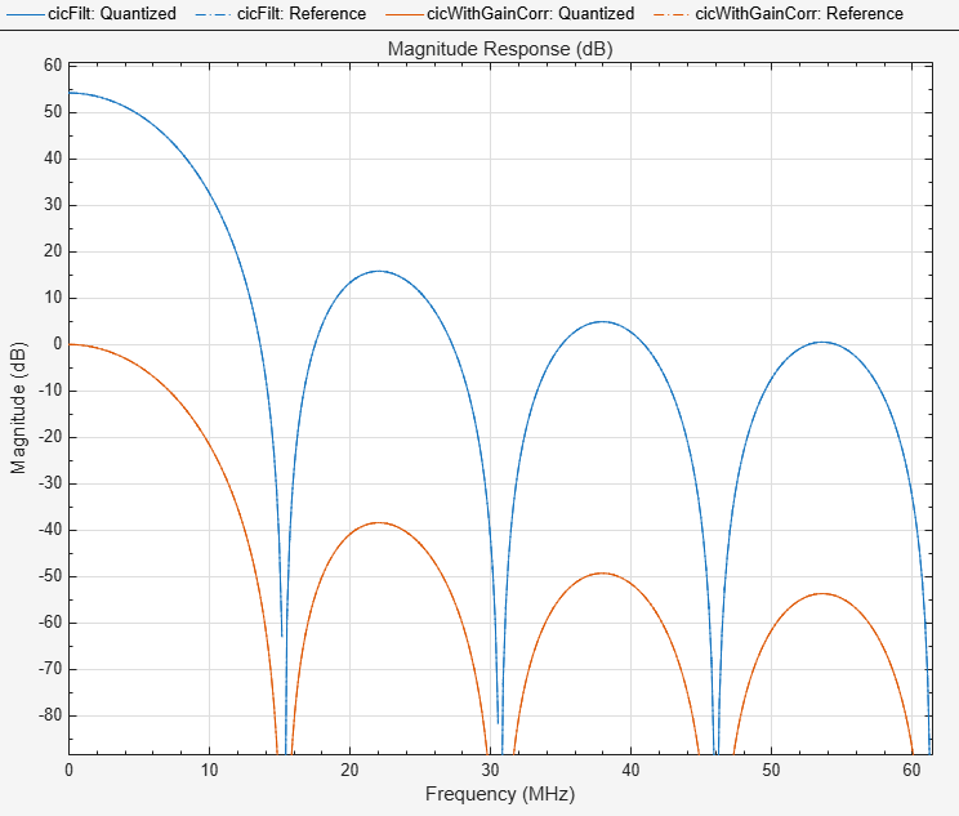CIC Droop Compensation Filter

The magnitude response of the CIC filter has a significant droop within the passband region, therefore an FIR-based droop compensation filter is used to flatten the passband response. The droop compensator is configured with the same parameters as the CIC decimator. This filter also implements decimation by a factor of two, therefore its bandlimiting characteristics are specified. Specify the filter requirements and then use the `design` function to return a filter System object with those characteristics.

```compParams.R = 2; % CIC compensation decimation factor compParams.Fpass = Fstop; % CIC comp passband frequency compParams.FsOut = cicParams.FsOut/compParams.R; % New sampling rate compParams.Fstop = compParams.FsOut - Fstop; % CIC comp stopband frequency compParams.Ap = Ap; % Same Ap as overall filter compParams.Ast = Ast; % Same Ast as overall filter compSpec = fdesign.decimator(compParams.R,'ciccomp',... cicParams.DifferentialDelay,... cicParams.NumSections,... cicParams.DecimationFactor,... 'Fp,Fst,Ap,Ast',... compParams.Fpass,compParams.Fstop,compParams.Ap,compParams.Ast,... cicParams.FsOut); compFilt = design(compSpec,'SystemObject',true) ```
```compFilt = dsp.FIRDecimator with properties: NumeratorSource: 'Property' Numerator: [-0.0398 -0.0126 0.2901 0.5258 0.2901 -0.0126 -0.0398] DecimationFactor: 2 Structure: 'Direct form' Use get to show all properties ```

Plot the combined response of the CIC filter (with gain correction) and droop compensation.

```ddcPlots.cicComp = fvtool(... dsp.FilterCascade(cicFilt,cicGainCorr,compFilt), ... 'Fs',FsIn,'Legend','off'); DDCTestUtils.setPlotNameAndTitle('CIC Decim + Droop Comp'); ```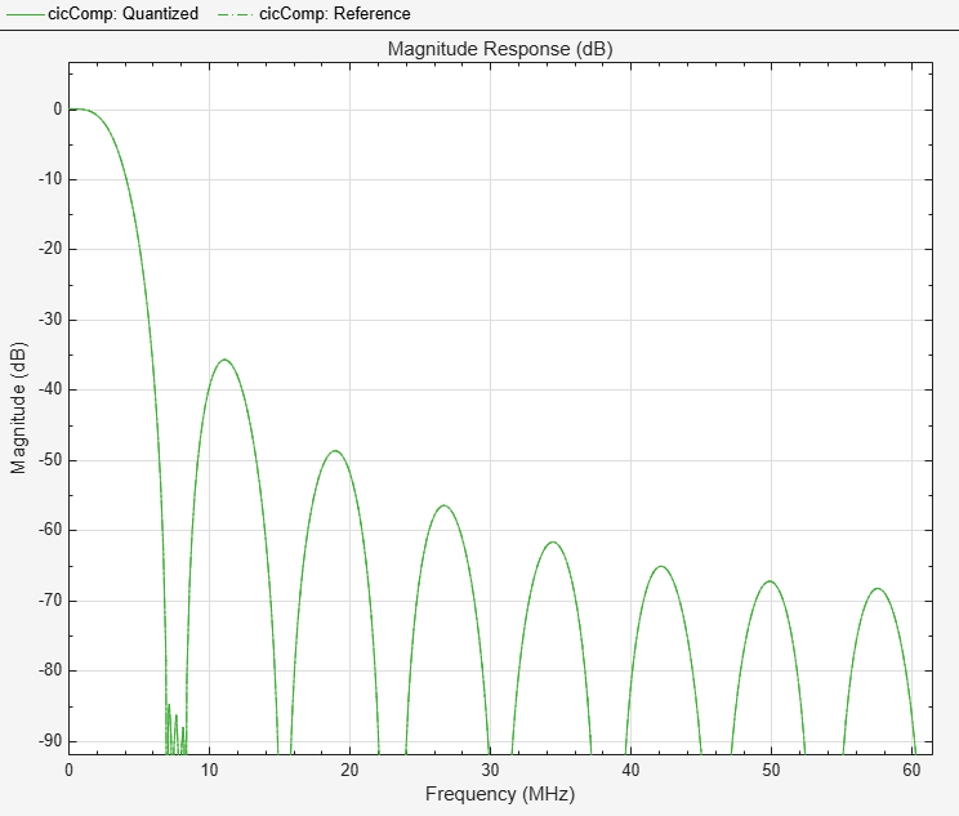Halfband Decimator

The halfband filter provides efficient decimation by two. Halfband filters are efficient because approximately half of their coefficients are equal to zero.

```hbParams.FsOut = compParams.FsOut/2; hbParams.TransitionWidth = hbParams.FsOut - 2*Fstop; hbParams.StopbandAttenuation = Ast; hbSpec = fdesign.decimator(2,'halfband',... 'Tw,Ast',... hbParams.TransitionWidth, ... hbParams.StopbandAttenuation,... compParams.FsOut); hbFilt = design(hbSpec,'SystemObject',true) ```
```hbFilt = dsp.FIRDecimator with properties: NumeratorSource: 'Property' Numerator: [1x11 double] DecimationFactor: 2 Structure: 'Direct form' Use get to show all properties ```

Plot the response of the DDC up to the halfband filter output.

```ddcPlots.halfbandFIR = fvtool(... dsp.FilterCascade(cicFilt,cicGainCorr,compFilt,hbFilt), ... 'Fs',FsIn,'Legend','off'); DDCTestUtils.setPlotNameAndTitle('CIC Decim + Droop Comp + HB FIR'); ```Final FIR Decimator

The final FIR implements the detailed passband and stopband characteristics of the DDC. This filter has more coefficients than the preceding FIR filters, however it operates at a lower sampling rate, which enables more resource sharing on hardware.

```% Add 3dB of headroom to the stopband attenuation so that the DDC still meets the % spec after fixed-point quantization. This value was determined by trial and error % with |fvtool|. finalSpec = fdesign.decimator(2,'lowpass',... 'Fp,Fst,Ap,Ast',Fpass,Fstop,Ap,Ast+3,hbParams.FsOut); finalFilt = design(finalSpec,'equiripple','SystemObject',true) ```
```finalFilt = dsp.FIRDecimator with properties: NumeratorSource: 'Property' Numerator: [1x70 double] DecimationFactor: 2 Structure: 'Direct form' Use get to show all properties ```

Visualize the overall magnitude response of the DDC.

```ddcFilterChain = dsp.FilterCascade(cicFilt,cicGainCorr,compFilt,hbFilt,finalFilt); ddcPlots.overallResponse = fvtool(ddcFilterChain,'Fs',FsIn,'Legend','off'); DDCTestUtils.setPlotNameAndTitle('Overall DDC Filter Chain'); ```### Fixed-Point Conversion

The frequency response of the floating-point DDC filter chain now meets the specification. Next, quantize each filter stage to use fixed-point types and analyze them to confirm that the filter chain still meets the specification.

Filter Quantization

This example uses 16-bit coefficients, which is sufficient to meet the specification. Using fewer than 18 bits for the coefficients minimizes the number of DSP blocks required for an FPGA implementation. The input to the DDC filter chain is 16-bit data with 15 fractional bits. The filter outputs are 18-bit values, which provides extra headroom and precision in the intermediate signals.

For the CIC decimator, choosing the `Minimum section word lengths` fixed-point data type option automatically optimizes the internal wordlengths based on the output wordlength and other CIC parameters.

```cicFilt.FixedPointDataType = 'Minimum section word lengths'; cicFilt.OutputWordLength = 18; ```

Configure the fixed-point parameters of the gain correction and FIR-based System objects. While not shown explicitly, the object uses the default `RoundingMethod` and `OverflowAction` settings (`Floor` and `Wrap` respectively).

```% CIC Gain Correction cicGainCorr.FullPrecisionOverride = false; cicGainCorr.CoefficientsDataType = 'Custom'; cicGainCorr.CustomCoefficientsDataType = numerictype(fi(cicGainCorr.Numerator,1,16)); cicGainCorr.OutputDataType = 'Custom'; cicGainCorr.CustomOutputDataType = numerictype(1,18,16); % CIC Droop Compensation compFilt.FullPrecisionOverride = false; compFilt.CoefficientsDataType = 'Custom'; compFilt.CustomCoefficientsDataType = numerictype([],16,15); compFilt.ProductDataType = 'Full precision'; compFilt.AccumulatorDataType = 'Full precision'; compFilt.OutputDataType = 'Custom'; compFilt.CustomOutputDataType = numerictype([],18,16); % Halfband hbFilt.FullPrecisionOverride = false; hbFilt.CoefficientsDataType = 'Custom'; hbFilt.CustomCoefficientsDataType = numerictype([],16,15); hbFilt.ProductDataType = 'Full precision'; hbFilt.AccumulatorDataType = 'Full precision'; hbFilt.OutputDataType = 'Custom'; hbFilt.CustomOutputDataType = numerictype([],18,16); % FIR finalFilt.FullPrecisionOverride = false; finalFilt.CoefficientsDataType = 'Custom'; finalFilt.CustomCoefficientsDataType = numerictype([],16,15); finalFilt.ProductDataType = 'Full precision'; finalFilt.AccumulatorDataType = 'Full precision'; finalFilt.OutputDataType = 'Custom'; finalFilt.CustomOutputDataType = numerictype([],18,16); ```

Fixed-Point Analysis

Inspect the quantization effects with `fvtool`. The filters can be analyzed individually, or in a cascade. `fvtool` shows the quantized and unquantized (reference) responses overlayed. For example, the effect of quantizing the final FIR filter stage is shown.

```ddcPlots.quantizedFIR = fvtool(finalFilt,'Fs',hbParams.FsOut,'arithmetic','fixed'); DDCTestUtils.setPlotNameAndTitle('Quantized Final Filter'); ```Redefine the `ddcFilterChain` cascade object to include the fixed-point properties of the individual filters. Then use `fvtool` to analyze the entire filter chain and confirm that the quantized DDC still meets the specification.

```ddcFilterChain = dsp.FilterCascade(cicFilt,cicGainCorr,compFilt,hbFilt,finalFilt); ddcPlots.quantizedDDCResponse = fvtool(ddcFilterChain,'Fs',FsIn,'Arithmetic','fixed'); DDCTestUtils.setPlotNameAndTitle('Quantized DDC Filter Chain'); legend(... 'DDC filter chain: Quantized', ... 'DDC filter chain: Reference'); ```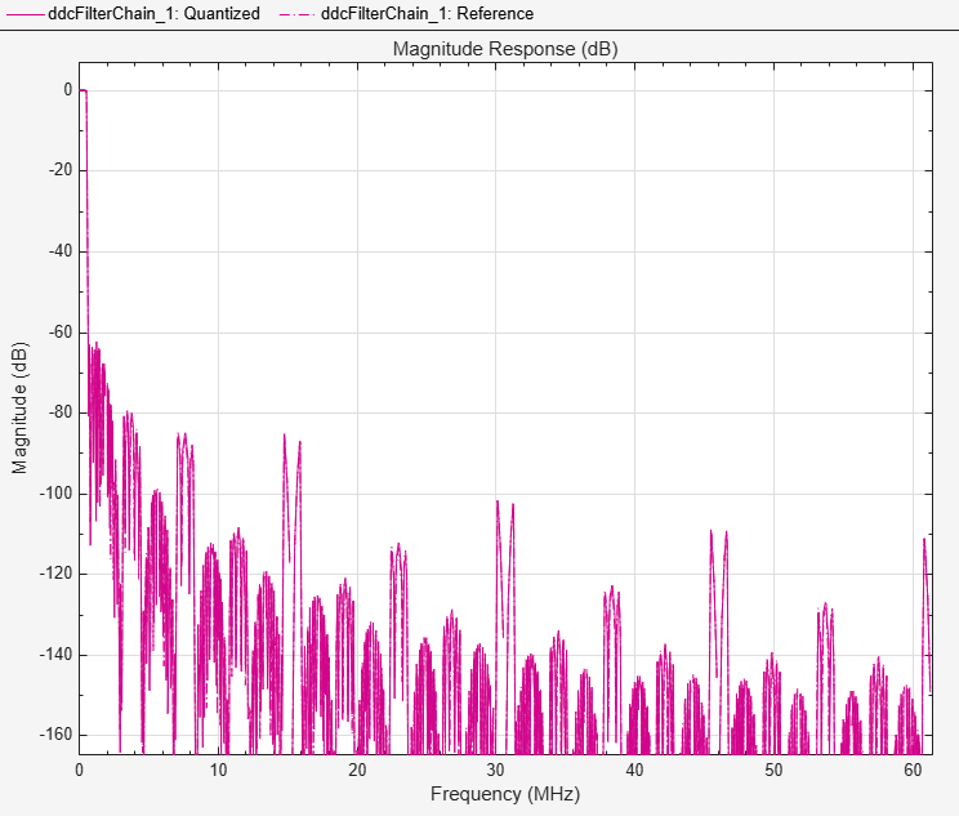### HDL-Optimized Simulink Model

The next step in the design flow is to implement the DDC in Simulink using HDL Coder compatible blocks.

Model Configuration

The model relies on variables in the MATLAB workspace to configure the blocks and settings. It uses the filter chain variables already defined. Next, define the Numerically Controlled Oscillator (NCO) parameters, and the input signal. These parameters are used to configure the NCO block.

Specify the desired frequency resolution. Calculate the number of accumulator bits required to achieve the desired resolution, and define the number of quantized accumulator bits. The quantized output of the accumulator is used to address the sine lookup table inside the NCO. Also compute the phase increment needed to generate the specified carrier frequency. Phase dither is applied to those accumulator bits which are removed during quantization.

```nco.Fd = 1; nco.AccWL = nextpow2(FsIn/nco.Fd) + 1; nco.QuantAccWL = 12; nco.PhaseInc = round((-Fc * 2^nco.AccWL)/FsIn); nco.NumDitherBits = nco.AccWL - nco.QuantAccWL; ```

The input to the DDC comes from `ddcIn`. For now, assign a dummy value for `ddcIn` so that the model can compute its data types. During testing, `ddcIn` provides input data to the model.

```ddcIn = 0; %#ok<NASGU> ```

### Model Structure

The top level of the DDC Simulink model is shown. The model imports `ddcIn` from the MATLAB workspace using a Signal From Workspace block, converts it to 16-bits and then applies it to the DDC. HDL code can be generated from the HDL_DDC subsystem.

```modelName = 'DDCHDLImplementation'; open_system(modelName); set_param(modelName,'SimulationCommand','Update'); set_param(modelName, 'Open','on'); ```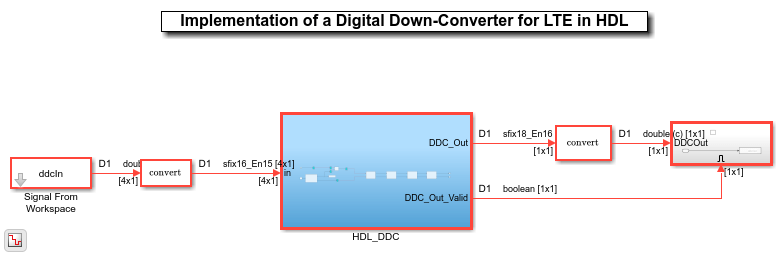The DDC implementation is inside the HDL_DDC subsystem. The NCO HDL Optimized block generates a complex phasor at the carrier frequency. This signal goes to a mixer which multiplies it with the input signal. The output of the mixer is then fed to the filter chain, where it is decimated to 1.92 Msps.

```set_param([modelName '/HDL_DDC'],'Open','on'); ```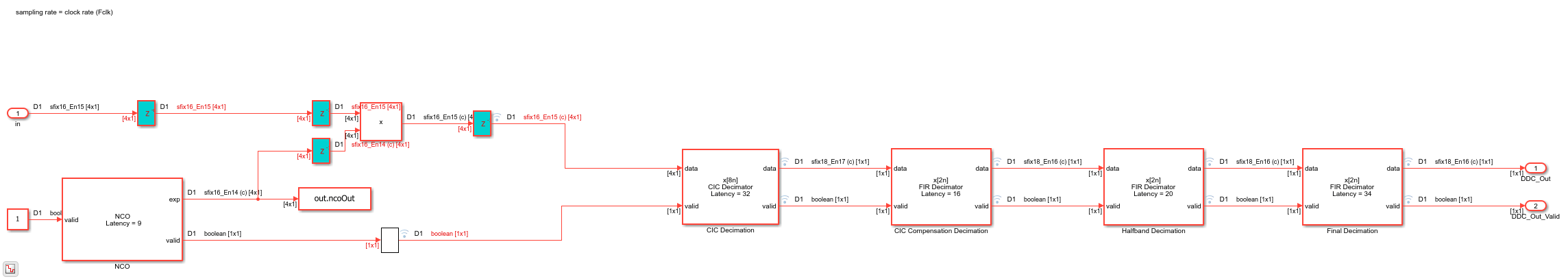NCO Block Parameters

The NCO block is configured with the parameters defined in the `nco` structure. Both tabs of the block's parameter dialog are shown.CIC Decimation and Gain Correction

The first filter stage is a Cascade Integrator-Comb (CIC) Decimator implemented with a CIC Decimation HDL Optimized block. The block parameters are set to the `cicParams` structure values. The gain correction is implemented by selecting the Gain correction parameter.

Filter Block Parameters

The filters are configured by using the properties of the corresponding System objects. The CIC Compensation, Halfband Decimation, and Final Decimation filters operate at effective sample rates that are lower than the clock rate (Fclk) by factors of 8, 16, and 32, respectively. These sample rates are implemented by using the valid signal to indicate which samples are valid at a particular rate. The signals in the filter chain all have the same Simulink sample time.

The CIC Compensation, Halfband Decimation, and Final Decimation filters are each implemented by a MATLAB Function Block and two Discrete FIR Filter HDL Optimized blocks in a polyphase decomposition. Polyphase decomposition implements the transform function, where,and. Polyphase decomposition is a resource-efficient way to implement decimation filters. The MATLAB Function Block holds two input samples every two cycles and passes them at the same time to the parallel pair of Discrete FIR Filter HDL Optimized blocksand. The lower subfilterand the upper subfiltereach contain half of the filter coefficients and process half of the input data.

For example, the CIC Compensation Decimation subsystem implements a `7`-coefficient filter. The upper subfilter,, has `4` coefficients and the lower subfilter,, has `3` coefficients. Each filter receives a sample and generates an output every `16` cycles. Because each filter processes one sample every 16 cycles, the subfilter blocks can share hardware resources in time. To optimize hardware resources in this way, both of the subfilters have the Filter structure parameter set to `Partly serial systolic`.

The diagram shows the CIC Compensation Decimation subsystem. The Halfband Decimation and the Final Decimation subsystems use the same structure.All the filter blocks are configured with the parameters defined in their corresponding structures. For example, the image shows the block parameters for the CIC Compensation Decimation block. The Number of cycles parameter is the minimum number of cycles between input samples. The input to the CIC Compensation Decimation block is sampled at `cicParams.DecimationFactor*compParams.R`, which is `16` cycles for both subfilters.The serial filter implementation reuses the multipliers in time over the number of clock cycles you specify. Without this optimization, the CIC Compensation Decimation filter with complex input data would use 14 multipliers. After the optimization, each ofanduses `2` multipliers for a total of `4`. Similarly, the Halfband Decimation and Final Decimation subsystems use `4` multipliers each.

### Sinusoid on Carrier Test and Verification

To test the DDC, modulate a 40kHz sinusoid onto the carrier frequency and pass it through the DDC. Then measure the Spurious Free Dynamic Range (SFDR) of the resulting tone and the SFDR of the NCO output.

```% Initialize random seed before executing any simulations. rng(0); % Generate a 40kHz test tone, modulated onto the carrier. ddcIn = DDCTestUtils.GenerateTestTone(40e3,Fc); % Demodulate the test signal with the floating point DDC. ddcOut = DDCTestUtils.DownConvert(ddcIn,FsIn,Fc,ddcFilterChain); release(ddcFilterChain); % Demodulate the test signal by executing the modified Simulink model with the sim function. out = sim(modelName); % Measure the SFDR of the NCO, floating point DDC and the fixed-point DDC outputs. results.sfdrNCO = sfdr(real(out.ncoOut),FsIn/64); results.sfdrFloatDDC = sfdr(real(ddcOut),FsIn/64); results.sfdrFixedDDC = sfdr(real(out.ddcOut),FsIn/64); disp('Spurious Free Dynamic Range (SFDR) Measurements'); disp([' Floating point DDC SFDR: ',num2str(results.sfdrFloatDDC) ' dB']); disp([' Fixed-point NCO SFDR: ',num2str(results.sfdrNCO) ' dB']); disp([' Optimized Fixed-point DDC SFDR: ',num2str(results.sfdrFixedDDC) ' dB']); fprintf(newline); % Plot the SFDR of the NCO and fixed-point DDC outputs. ddcPlots.ncoOutSDFR = figure; sfdr(real(out.ncoOut),FsIn/64); DDCTestUtils.setPlotNameAndTitle(['NCO Out ' get(gca,'Title').String]); ddcPlots.OptddcOutSFDR = figure; sfdr(real(out.ddcOut),FsIn/64); DDCTestUtils.setPlotNameAndTitle(['Optimized Fixed-Point DDC Out ' get(gca,'Title').String]); ```
```Spurious Free Dynamic Range (SFDR) Measurements Floating point DDC SFDR: 291.3483 dB Fixed-point NCO SFDR: 83.0249 dB Optimized Fixed-point DDC SFDR: 108.8419 dB ```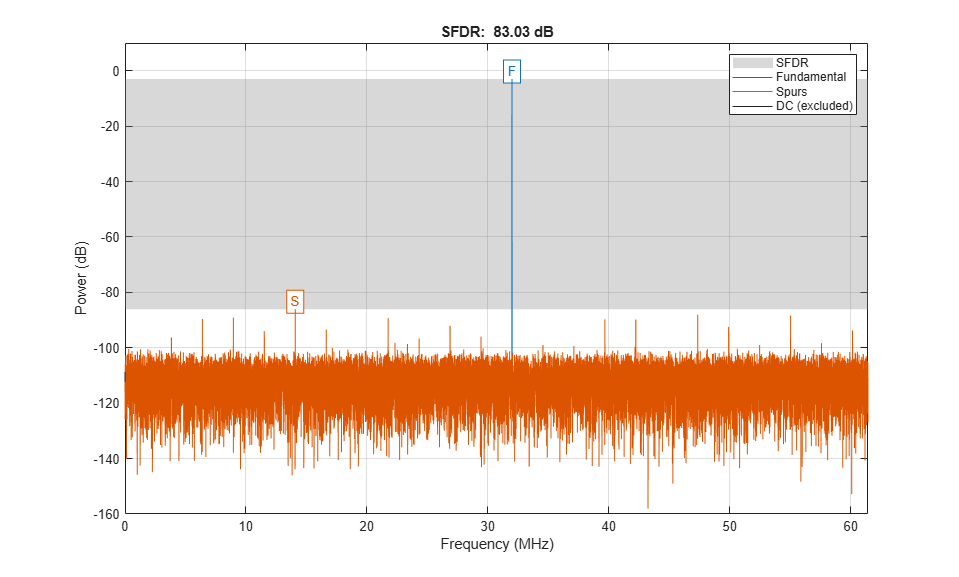### LTE signal test

```rng(0); if license('test','LTE_Toolbox') % Generate a modulated LTE test signal with LTE Toolbox [ddcIn, sigInfo] = DDCTestUtils.GenerateLTETestSignal(Fc); % Downconvert with a MATLAB Floating Point Model ddcOut = DDCTestUtils.DownConvert(ddcIn,FsIn,Fc,ddcFilterChain); release(ddcFilterChain); % Downconvert using Simulink model ddcIn=[ddcIn;zeros(320,1)]; % Adding zeros to make up propagation latency to output complete result out = sim(modelName); results.evmFloat = DDCTestUtils.MeasureEVM(sigInfo,ddcOut); results.evmFixed = DDCTestUtils.MeasureEVM(sigInfo,out.ddcOut); disp('LTE Error Vector Magnitude (EVM) Measurements'); disp([' Floating point DDC RMS EVM: ' num2str(results.evmFloat.RMS*100,3) '%']); disp([' Floating point DDC Peak EVM: ' num2str(results.evmFloat.Peak*100,3) '%']); disp([' Fixed-point HDL Optimized DDC RMS EVM: ' num2str(results.evmFixed.RMS*100,3) '%']); disp([' Fixed-point HDL Optimized DDC Peak EVM: ' num2str(results.evmFixed.Peak*100,3) '%']); fprintf(newline); end ```
```LTE Error Vector Magnitude (EVM) Measurements Floating point DDC RMS EVM: 0.633% Floating point DDC Peak EVM: 2.44% Fixed-point HDL Optimized DDC RMS EVM: 0.731% Fixed-point HDL Optimized DDC Peak EVM: 2.69% ```

### HDL Code Generation and FPGA Implementation

To generate the HDL code for this example you must have the HDL Coder™ product. Use the `makehdl` and `makehdltb` commands to generate HDL code and an HDL testbench for the HDL_DDC subsystem. The DDC was synthesized on a Xilinx® Zynq®-7000 ZC706 evaluation board. The post place-and-route resource utilization results are shown in the table. The design met timing with a clock frequency of 313 MHz.

```T = table(... categorical({'LUT'; 'LUTRAM'; 'FF'; 'BRAM'; 'DSP'}),... categorical({'2660'; '318'; '5951'; '1.0'; '18'}),... 'VariableNames',{'Resource','Usage'}) ```
```T = 5x2 table Resource Usage ________ _____ LUT 2660 LUTRAM 318 FF 5951 BRAM 1.0 DSP 18 ```

## Support

#### Deploying 5G NR Wireless Communications on FPGAs: A Complete MATLAB and Simulink Workflow

Download white paper